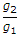# Civil Engineering - Applied Mechanics

### Exercise :: Applied Mechanics - Section 1

26.

A square hole is punched out of a circular lamina, the diagonal of the square being the radius of the circle. If r is the radius of the circle, the C.G. of the remainder from the corner of the square on the circumference will be

 A.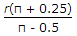B.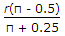C.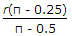D.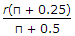Explanation:

No answer description available for this question. Let us discuss.

27.

A bullet weighing 200 g is fired horizontally with a velocity of 25 m/sec from a gun carried on a carriage which together with the gun weighs 100 kg. The velocity of recoil of the gun, will be

 A. 0.01 m/sec B. 0.05 m/sec C. 1.00 m/sec D. 1.5 m/see.

Explanation:

No answer description available for this question. Let us discuss.

28.

The tension in a cable supporting a lift

 A. is more when the lift is moving downwards B. is less when the lift is moving upwards C. remains constant whether its moves downwards or upwards D. is less when the lift is moving downwards.

Explanation:

No answer description available for this question. Let us discuss.

29.

A satellite moves in its orbit around the earth due to

 A. Gravitational force B. Centripetal force C. Centrifugal force D. none of these.

Explanation:

No answer description available for this question. Let us discuss.

30.

If g1 and g2 are the gravitational accelerations on two mountains A and B respectively, the weight of a body when transported from A to B will be multiplied by

 A. g1 B. g2 C.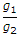D.